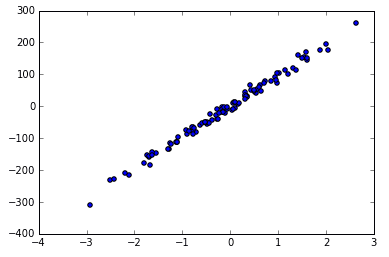# sklearn 入门笔记

## 什么是机器学习？

sklearn 给了一个定义

In general, a learning problem considers a set of n samples of data and then tries to predict properties of unknown data. If each sample is more than a single number and, for instance, a multi-dimensional entry (aka multivariate data), it is said to have several attributes or features.

### 要解决的问题

1. Classification 分类，也就是离散的
2. Regression 回归，也就是连续的
3. Clustering 聚类
4. Dimensionality reduction 数据降维

1. Model Selection 模型选择
2. Preprocessing 数据预处理## sklearn 的数据库

sklearn 为了方便学习自带了一些数据库，可以说是非常方便了。包括了 iris 花瓣数据库，手写数字数据库等。这些例子可以说相当于编程语言的 hello world 或者是图形学届的 utah teapot 了。

>>> from sklearn import datasets
>>> iris = datasets.load_iris()          # iris 花瓣数据库
>>> digits = datasets.load_digits()      # 手写数字数据库
>>> print(digits.data)                   # 数据库的输入
[[  0.   0.   5. ...,   0.   0.   0.]
[  0.   0.   0. ...,  10.   0.   0.]
[  0.   0.   0. ...,  16.   9.   0.]
...,
[  0.   0.   1. ...,   6.   0.   0.]
[  0.   0.   2. ...,  12.   0.   0.]
[  0.   0.  10. ...,  12.   1.   0.]]
>>> digits.target                        # 数据库的输出
array([0, 1, 2, ..., 8, 9, 8])


## 学习与预测

>>> from sklearn import svm
>>> classifier = svm.SVC(gamma=0.001, C=100.)

>>> classifier.fit(digits.data[:-1], digits.target[:-1])  # 注意第一个参数是数据，第二个参数是结果
SVC(C=100.0, cache_size=200, class_weight=None, coef0=0.0,
decision_function_shape="ovr", degree=3, gamma=0.001, kernel="rbf",
max_iter=-1, probability=False, random_state=None, shrinking=True,
tol=0.001, verbose=False)

# 现在我们开始预测最后一个数据
>>> classifier.predict(digits.data[-1:])
array()  # 得出的结果是 8from sklearn.model_selection import train_test_split

X_train, X_test, y_train, y_test = train_test_split(
iris_X, iris_y, test_size=0.3)

# 注意其中训练数据被 sklearn 打乱了。在机器学习中，数据比较乱是比较好的，算法其实也一样，数组是乱的最好。


### 保存模型

>>> from sklearn.externals import joblib
>>> joblib.dump(classifier, "filename.pkl")



## 其他的一些技巧

### 一些约定

1. 所有的输入都会被转化为 float64 类型
2. 一半习惯用 X 表示样本数据，y 表示预测结果

### 可视化

>>> X, y = datasets.make_regression(n_samples=100, n_features=1, n_targets=1, noise=10)
>>> plt.scatter(X, y)
>>> plt.show()## 参考

1. https://sklearn.apachecn.org/docs/0.21.3/2.html
2. https://morvanzhou.github.io/tutorials/machine-learning/sklearn/1-1-why/
3. http://scikit-learn.org/stable/tutorial/basic/tutorial.html

#### 及时获取更新，请关注公众号“爬虫技术学习”(spider-learn)